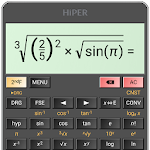ID: cz.hipercalc

The description of HiPER Scientific Calculator

HiPER Scientific Calculator is a popular calculator with more than 25 million downloads and 100 000 five-star ratings.

The calculator has up to 100 digits of significand and 9 digits of exponent. It detects repeating decimals and numbers can be also entered as fractions or converted to fractions.

You can write expressions in a natural way and watch your calculations. The result is displayed as a number, simplified expression etc.

The calculator has several layouts suitable for various screen sizes:
- \"pocket\" for small devices
- \"compact\" for smartphones (in portrait and landscape orientation)
- \"expanded\" for tablets

A multiline display can be turned on in tablets to show the complete history of calculations and to provide access the previous results.

Users can choose from several high-quality themes.

The calculator has many functions, such as:
- basic arithmetic operations including percentage, modulo and negation;
- fractions (in the expression mode any expression including nested fractions can be entered as a numerator and a denominator);
- mixed numbers;
- periodic numbers and their conversion to fractions;
- unlimited number of braces;
- operator priority;
- repeated operations;
- equations
- variables and symbolic computation;
- derivatives and integrals;
- graphs of functions and integral area, 3D graphs;
- calculation details - extended information about a calculation like all complex roots, unit circle etc.;
- complex numbers
- conversion between rectangular and polar coordinates
- sums and products of sequences
- advanced number operations such as random numbers, combinations, permutations, common greatest divisor, etc.;
- trigonometric and hyperbolic functions;
- powers, roots, logarithms, etc.;
- degrees, minutes and seconds conversion;
- fixed point, scientific and engineering display format;
- display exponent as SI units prefix;
- memory operations with 10 extended memories;
- clipboard operations with various clipboard formats;
- result history;
- binary, octal and hexadecimal numeral systems;
- logical operations;
- bitwise shifts and rotations;
- haptic feedback;
- more than 90 physical constants;
- conversion among 250 units;
- Reverse Polish notation.

The calculator has many settings to manage the full screen mode, decimal and thousand separators, etc.

All features are described with a built-in help.

HiPER Scientific Calculator 9.1.2 APK for Android 4.1+

 Version 9.1.2 for Android 4.1+ Update on 2022-01-15 Installs 10.000.000++ File size 8.559.884 bytes Permissions view permissions What's new - Statistical functions- New detail: Statistics- Equations with matrices- Matrix inverse added to MTRX menu- >BASE function available in custom keyboards- Improved equations solving numerical method- Complex menu key available in custom keyboards- "Division with remainders" detail displayed also for polynomials- Delta percentage function- HISTORY key added to variable value editing

FAQs

• What is HiPER scientific calculator?
Simple business calculator is a small Android app, like a normal calculating device used to perform both basic and complex arithmetic operations.
• Which is best app for scientific calculator?
Top 8 Best Android Calculator Apps – 2019. Google Calculator. Google's Calculator application is one of the more simpler ones on this list. ... RealCalc Scientific Calculator. If you need something more advanced, RealCalc is a great option. ... CALCU. ... HiPER Scientific Calculator. ... Mobi Calculator. ... All-In-One Calculator.
• Is there any app for scientific calculator?
List of Scientific calculator App IOS and Android: ... HiPER Scientific Calculator. RealCalc Scientific Calculator. Scientific Calculator free.